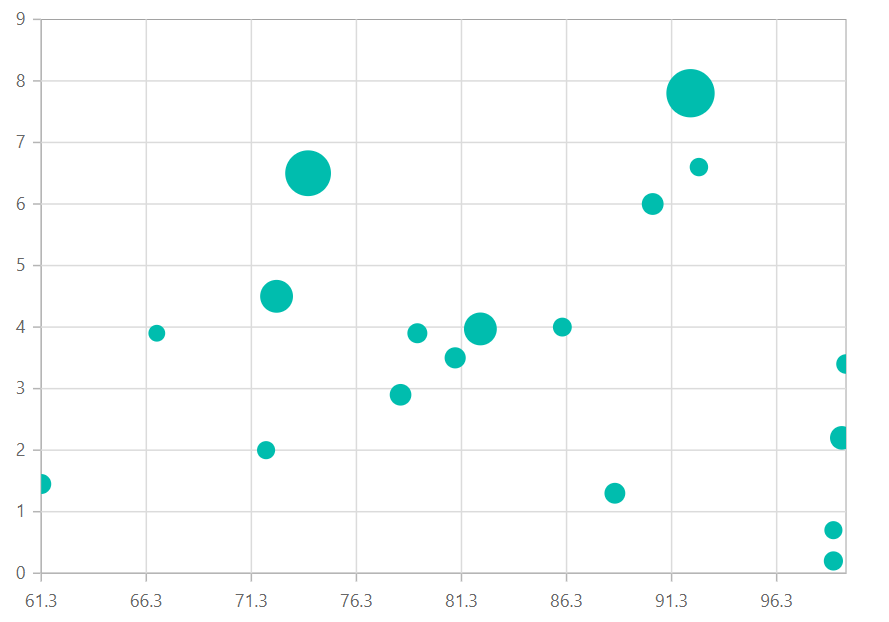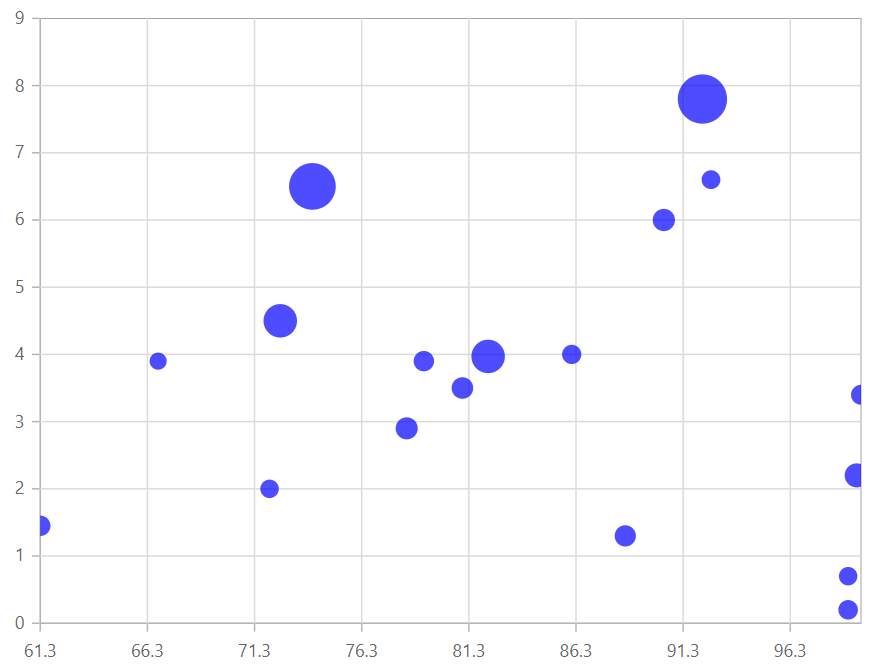# Bubble in Blazor Charts Component

11 Aug 20235 minutes to read

## Bubble

Bubble Chart is similar to the Scatter chart but it also visualizes the third parameter by its size. To render a bubble series, set series Type as Bubble. It visualizes data with three parameters such as XName, YName, and Size. The bubble size depends on third parameter.

NOTE

Refer to our Blazor Bubble Charts feature tour page to know about its other groundbreaking feature representations and also explore our Blazor Bubble Chart Example to know how to render and configure the bubble type charts.

## Size mapping

The Size property can be used to map the size value specified from datasource.

``````@using Syncfusion.Blazor.Charts

<SfChart>
<ChartSeriesCollection>
<ChartSeries DataSource="@SalesReports" XName="X" YName="Y" Type="ChartSeriesType.Bubble">
</ChartSeries>
</ChartSeriesCollection>
</SfChart>

@code{
public class ChartData
{
public double X { get; set; }
public double Y { get; set; }
public string Text { get; set; }
}

public List<ChartData> SalesReports = new List<ChartData>
{
new ChartData { X= 92.2, Y= 7.8, Text= "China" },
new ChartData { X= 74, Y= 6.5, Text= "India" },
new ChartData { X= 90.4, Y= 6.0, Text= "Indonesia" },
new ChartData { X= 99.4, Y= 2.2, Text= "US" },
new ChartData { X= 88.6, Y= 1.3, Text= "Brazil" },
new ChartData { X= 99, Y= 0.7, Text= "Germany" },
new ChartData { X= 72, Y= 2.0, Text= "Egypt" },
new ChartData { X= 99.6, Y= 3.4, Text= "Russia" },
new ChartData { X= 99, Y= 0.2, Text= "Japan" },
new ChartData { X= 86.1, Y= 4.0, Text= "Mexico" },
new ChartData { X= 92.6, Y= 6.6, Text= "Philippines" },
new ChartData { X= 61.3, Y= 1.45, Text= "Nigeria" },
new ChartData { X= 82.2, Y= 3.97, Text= "Hong Kong" },
new ChartData { X= 79.2, Y= 3.9, Text= "Netherland" },
new ChartData { X= 72.5, Y= 4.5, Text= "Jordan" },
new ChartData { X= 81, Y= 3.5, Text= "Australia" },
new ChartData { X= 66.8, Y= 3.9, Text= "Mongolia" },
new ChartData { X= 78.4, Y= 2.9, Text= "Taiwan" }
};
}``````## Series customization

The following properties can be used to customize the Bubble series.

• Fill – Specifies the color of series.
• Opacity – Specifies the opacity of Fill.
``````@using Syncfusion.Blazor.Charts

<SfChart>
<ChartSeriesCollection>
<ChartSeries DataSource="@SalesReports" XName="X" YName="Y" Opacity="0.7" Fill="blue" Size="Size" Type="ChartSeriesType.Bubble">
</ChartSeries>
</ChartSeriesCollection>
</SfChart>

@code{
public class ChartData
{
public double X { get; set; }
public double Y { get; set; }
public double Size { get; set; }
public string Text { get; set; }
}

public List<ChartData> SalesReports = new List<ChartData>
{
new ChartData { X= 92.2, Y= 7.8, Size= 1.347, Text= "China" },
new ChartData { X= 74, Y= 6.5, Size= 1.241, Text= "India" },
new ChartData { X= 90.4, Y= 6.0, Size= 0.238, Text= "Indonesia" },
new ChartData { X= 99.4, Y= 2.2, Size= 0.312, Text= "US" },
new ChartData { X= 88.6, Y= 1.3, Size= 0.197, Text= "Brazil" },
new ChartData { X= 99, Y= 0.7, Size= 0.0818, Text= "Germany" },
new ChartData { X= 72, Y= 2.0, Size= 0.0826, Text= "Egypt" },
new ChartData { X= 99.6, Y= 3.4, Size= 0.143, Text= "Russia" },
new ChartData { X= 99, Y= 0.2, Size= 0.128, Text= "Japan" },
new ChartData { X= 86.1, Y= 4.0, Size= 0.115, Text= "Mexico" },
new ChartData { X= 92.6, Y= 6.6, Size= 0.096, Text= "Philippines" },
new ChartData { X= 61.3, Y= 1.45, Size= 0.162, Text= "Nigeria" },
new ChartData { X= 82.2, Y= 3.97, Size= 0.7, Text= "Hong Kong" },
new ChartData { X= 79.2, Y= 3.9, Size= 0.162, Text= "Netherland" },
new ChartData { X= 72.5, Y= 4.5, Size= 0.7, Text= "Jordan" },
new ChartData { X= 81, Y= 3.5, Size= 0.21, Text= "Australia" },
new ChartData { X= 66.8, Y= 3.9, Size= 0.028, Text= "Mongolia" },
new ChartData { X= 78.4, Y= 2.9, Size= 0.231, Text= "Taiwan" }
};
}``````NOTE

Refer to our Blazor Charts feature tour page for its groundbreaking feature representations and also explore our Blazor Chart Example to know various chart types and how to represent time-dependent data, showing trends at equal intervals.